# 3.6 Approximation of the Self-Energy

An exact evaluation of the self-energy is possible only for some rather pathological cases. For real systems one has to rely on approximation schemes. Hence, a natural approach is to retain the single-particle picture and assume that each particle moves in a single-particle potential that comes from its average interaction with all of the other particles. Thus, as a first-order approximation one can keep just the first-order contribution to the proper self-energy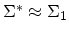(see Fig. 3.7). This approximation corresponds to summing an infinite class of diagrams containing arbitrary iterations of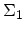. Therefore, any approximation for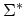generates an infinite-order series for the GREEN's function (see Fig. 3.5).

However, using non-interacting GREEN's function in self-energies, which is referred to as BORN approximation, is not fully consistent, since the background particles contributing to the self-energy are treated as non-interacting. In reality, of course, these particles also move in an average potential coming from the presence of all the other particles. Thus instead of non-interacting GREEN's functions (single lines), one has to use the exact GREEN's function (double line) in the proper self-energy, as shown in Fig. 3.9. Since the exact GREEN's function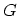both determines and is determined by the proper self-energy, this approximation is known as the self-consistent BORN approximation (see Appendix F.3). The self-consistent approach preserves conservation laws (Appendix F.3), for example, the continuity equation holds valid (Section 3.9.4). Throughout this work the self-consistent BORN approximation is applied.

Figure 3.9: FEYNMAN diagrams of the self-consistent first-order proper self-energies.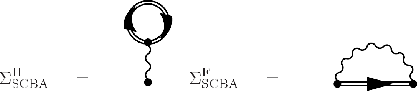Subsections

M. Pourfath: Numerical Study of Quantum Transport in Carbon Nanotube-Based Transistors